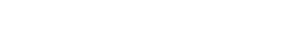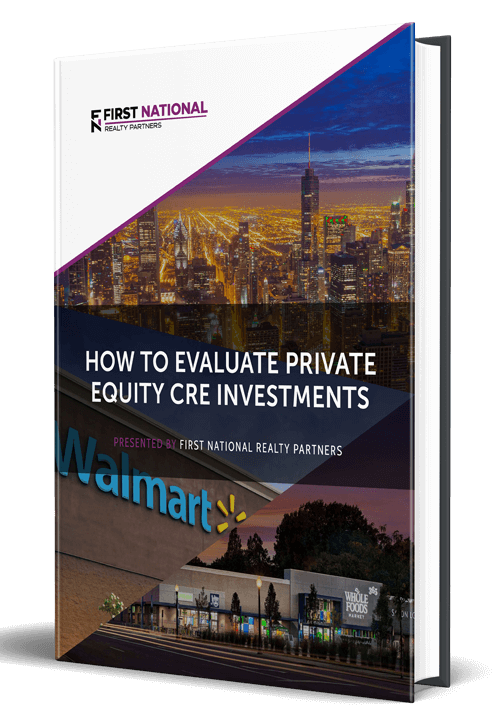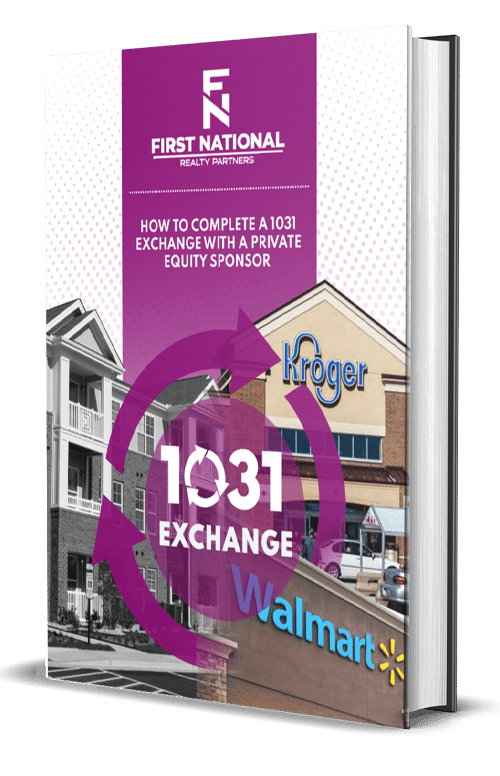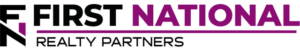# Equity Dividend Rate In Real Estate: An Investor’s Guide

### Key Takeaways

• The equity dividend rate is a commercial real estate return metric that describes the relationship between the cash received by an investor in a given year relative to the amount of cash invested.  It represents the annual return on investment.
• The formula used to calculate the equity dividend rate is cash received in a year divided by the total cash investment.  For example, if a property owner receives \$10,000 in a year on a \$100,000 cash investment, the resulting equity dividend rate is 10%.
• A good equity dividend rate is relative to an investor’s unique return requirements, but somewhere in the range of 6% – 12% is generally considered to be good.
• Finally, the equity dividend rate goes by another name, the cash on cash return, but the formula and the meaning of the result is the same.

When a real estate investor purchases a property, they rarely do so with cash.  Instead, they get a loan (debt) for some percentage of the purchase price (typically 60% – 80%) and use their own capital (equity) to make up the difference.  While investors like to measure the return for the entire transaction (debt and equity), they also like to know the return on just the cash that they invested.  This is measured using a metric called the equity dividend rate.

In this article, we will define what the equity dividend rate is, how it is calculated in a real estate transaction, and why it is important.  By the end, readers will be familiar with this metric and should be able to calculate it as part of their own pre-purchase due diligence process.

At First National Realty Partners, we use the equity dividend rate as a way to identify the deals that have the most potential profit for our investors.  To learn more about our current investment opportunities, click here.

## What is the Equity Dividend Rate?

The equity dividend rate is a commercial real estate performance metric that measures an investor’s annual return on the amount of cash invested in a property.  Along with the cap rate, the equity dividend rate is one of the most common metrics used to measure commercial real estate investment profitability.

## How to Calculate the Equity Dividend Rate

In real estate investing, the equity dividend rate is calculated as the ratio of the cash received in a given year to the total cash invested.  The equity dividend rate formula looks like this:

• Equity Dividend Rate = Annual Cash Received / Total Cash Invested

### Components of the Equity Dividend Rate

To illustrate how this formula works, it is helpful to break it down into its components.

The annual cash received represents the income received by an investor in one year on either a “levered” or “unlevered” basis.  If it is calculated on a levered basis, the annual cash received is represented by the amount of money left after a property’s debt service has been paid (pre-tax cash flow).  On an unlevered basis, the annual cash flow is the same as the property’s net operating income, which is calculated as effective gross income less operating expenses (property taxes, maintenance, insurance, etc).

#### Total Cash Invested

The total cash invested is represented by the amount of money that a real estate investor puts into a deal – the equity investment.  On a levered basis, total cash invested is equal to the down payment on the property.  On an unlevered basis, this is equal to the purchase price of the property, because it was purchased in cash.

### Example of Levered & Unlevered Equity Dividend Rate

To illustrate the difference between the levered and unlevered equity dividend rate, consider a property with the following attributes:

• Net Operating Income (NOI):  \$500,000
• Cash Flow After Debt Service:  \$250,000
• Purchase Price:  \$5,000,000
• Down Payment:  \$1,000,000

Based on these inputs, we can calculate the following equity dividend rates:

 Unlevered Levered Numerator: \$500,000 \$250,000 Denominator: \$5,000,000 \$1,000,000 Result: 10.00% 25.00%

On the unlevered side, the numerator in the equation is net operating income (NOI) and the denominator is the purchase price, because unlevered means that the property was purchased with cash.  The result is a 10% annual rate of return, which is equivalent to the capitalization rate.

On the levered side, the numerator is the cash flow after debt service and the denominator is the down payment on the investment property.  The result is 25%, which demonstrates the boost in returns that debt can provide to a property.  So, these results beg the question, what is a good equity dividend rate for a commercial real estate property?

## What is a Good Equity Dividend Rate?

The idea of a “good” equity dividend rate for commercial real estate is somewhat subjective.  As a general rule of thumb, a good equity dividend rate ranges from 6% – 12% annually.  But, this range is also unique to each individual investor who has their own objectives.  For example, a 10% equity dividend rate could be considered “good,” but if an investor needs to meet a threshold of 15%, this may not be so good after all.

So, each real estate investor should set their own desired equity dividend rate range and evaluate the quality of the calculated result against it.

## Equity Dividend Rate vs. Cash on Cash Return

It is worth noting that the equity dividend rate also goes by another name, the cash on cash return.  The formula is the same and the measured result provides the same information regardless of property type (office building, multifamily, industrial, etc).

## Summary & Conclusions

The equity dividend rate is a commercial real estate return metric that describes the relationship between the cash received by an investor in a given year relative to the amount of cash invested.  It represents the annual return on investment on the cash invested.

The formula used to calculate the equity dividend rate is cash received in a year divided by the total cash investment.  For example, if a property owner receives \$10,000 in a year on a \$100,000 cash investment, the resulting equity dividend rate is 10%.

A good equity dividend rate is relative to a real estate investor’s unique return requirements.  But, an equity dividend rate in the range of 6% – 12% is generally considered to be good.

Finally, the equity dividend rate goes by another name, the cash on cash return, but the formula and the meaning of the result is the same.

## Interested In Learning More?

First National Realty Partners is one of the country’s leading private equity commercial real estate investment firms. With an intentional focus on finding world-class, multi-tenanted assets well below intrinsic value, we seek to create superior long-term, risk-adjusted returns for our investors while creating strong economic assets for the communities we invest in.

Get instant access to all of our current and past commercial real estate deals.## Subscribe Now

Get the latest news on real estate

FNRP Spotlight Series

1031 Exchange

Acquisitions

## Get More From FNRP## How to Evaluate Private Equity CRE Investments## How to Complete a 1031 Exchange with a Private Equity Sponsor